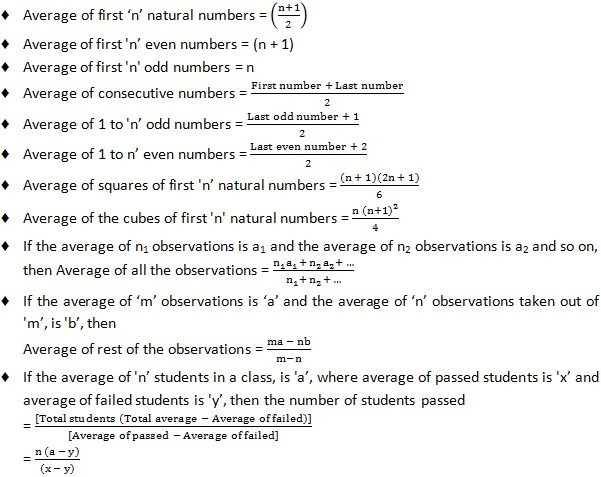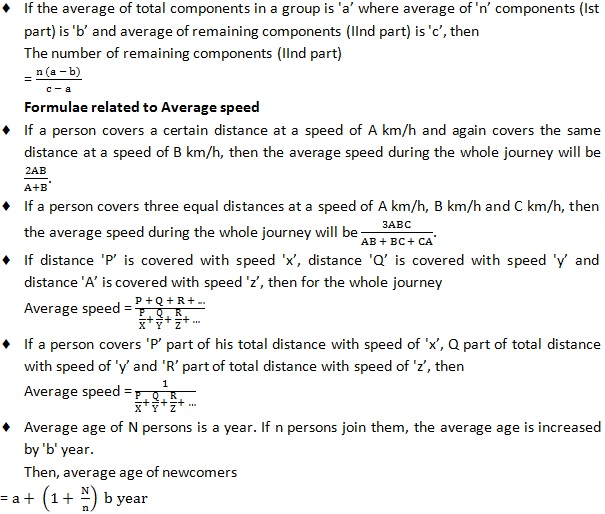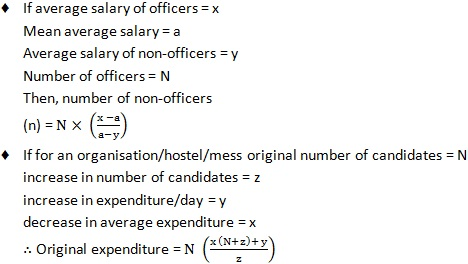# Quantitative Aptitude: Averages: List of Formulas for speedy calculations

Jagranjosh.com experts has come up with Important formulas of Quantitative Aptitude (Averages)t through which the aspirants can get help in Banking, SSC, Railway and other competitive exams.

Jagranjosh.com team always tries on new methods through which the aspirants can get help in their exams. Here, we are providing the candidates a set of formulas for the Quantitative Aptitude section. Candidates who are preparing for Banking and SSC exams can easily learn the formulas as the entire set of formulas are assembled together. Practice can be easier and calculations faster can increase the probability to crack the Quantitative Aptitude Section in any Banking and SSC exam. This is an attempt to help the candidates preparing for the Banking, SSC, Railway and other competitive exams.We have arrived in with the formulas for Averages chapter, which will sure make calculations speedy. The section is divided into points to remember and formulas to remember for trouble-free learning.

Averages: Important Points and Formulas

Points To Remember:

• If all the numbers are increased by 'a’, then their average will also be increased by 'a’.
• If all the numbers are decreased by ‘a’, then their average will also be decreased by 'a’.
• If all the numbers are multiplied by 'a’, then their average will also be multiplied by 'a'.
• If all the numbers are divided by 'a', then their average will also be divided by 'a’.

Formulae To Remember: